# Equilibrium Calculation and Technological Parameters Optimization of Natural Gas Liquefaction Process with Mixed Refrigerant

Equilibrium Calculation and Technological Parameters Optimization of Natural Gas Liquefaction Process with Mixed Refrigerant

S. Li Y. D. Zhang* Y. Li R. Q. Liao*

Key Laboratory of Oil Gas Production, Research Center of Yangtze University and China National Petroleum Corporation, Yangtze University, Wuhan, Hubei 430100, China;

State Key Laboratory of Coal Combustion, Huazhong University of Science and Technology, Wuhan, Hubei 430074, China;

Gas Lift Technology Center of Tuha Oilfield of China National Petroleum Corporation, Hami 838202, Xinjiang Province, P.R. China.

Corresponding Author Email:
yindi.zhanghust@gmail.com; liaoruiquan@163.com.
Page:
123-128
|
DOI:
https://doi.org/10.18280/ijht.3302120
N/A
|
Accepted:
N/A
|
Published:
30 June 2015
| Citation

OPEN ACCESS

Abstract:

Mixed-Refrigerant Cycle (MRC) is widely used in natural gas liquefaction. In the process, phase equilibrium of raw natural gas and mixture refrigerant is the basis of thermodynamics analysis and liquefaction-process simulation. This paper calculates the vapor-liquid equilibrium of mixture by using the SRK and PR equation of state. The results show the PR equation has higher precision. And on this basis, this paper analyzes the impacts of mixture components, pressure and temperature on equilibrium constant. There is a conclusion that a rational reducing of lighter hydrocarbons, increasing pressure and decreasing temperature can increase the liquid ration, which can help to obtain an optimal vapor-liquid equilibrium, reinforce the cooling effectiveness, and improve liquefaction efficiency.

Keywords:

Natural gas, Phase equilibrium, Equation of state, Equilibrium constant, optimization analysis.

1. Introduction

Vapor-liquid equilibrium (VLE) calculation is a basic work for natural gas industries. In cascade liquefaction process, mixing refrigerant liquefaction process and liquefaction with expander, mixing refrigerant is the most widely used of three [1-3]. In this process, after cooling of the compressed mixture refrigerant enters the gas-liquid separator, the liquid-phase supplies cold energy for heat-exchanger, and raw natural gas and gas-phase refrigerant are cooled. During a series of thermodynamic processes, the temperature, pressure and gas-liquid phase distribution of mixture system are changed[4-7]. It all goes back to the vapor-liquid equilibrium. Then accurately understanding for phase proportion of mixture is the key issue for calculation of thermodynamics properties, and can reveal the liquefaction degree, cooling effectiveness and resource consumption. Hence, phase state and components distribution of mixture is the key of design, operation and optimization of process [8-10].

In the liquefied process, the vapor-liquid phase constants can describe the vapor-liquid distribution clearly. Make an understanding and analysis for the impact factors on equilibrium constant is helpful for refrigerant choice and components proportion. In currently, the researches of equilibrium are focused on the equilibrium calculation with EOS methods and improvement of equation [8-12]. And little research has been done on process optimization from the point of vapor-liquid equilibrium view. Under this background, this paper calculates the phase equilibrium of natural gas with simple components by using cubic equation and analyzes the influence parameters, which provides some reliable basis for designing and optimizing of natural gas liquefaction.

2. Phase Equilibrium Calculation

Equation of state is anticipated to become the major way to calculate equilibrium. And cubic equation with two constants are used extensively in the chemistry, petrochemical, and natural gas industries because of their relatively simplicity, convenience and accuracy. Especially modification of the Redlich-Kwong equation that proposed by Soave  and Peng-Robinson equation  have great advantages in phase equilibrium calculation of mixture of hydrocarbons.

2.1. Equation of state model

RKS equation of state and mixing rules.

The general form of that cubic EOS is as follows :

$p=\frac{R T}{V_{m}-b}-\frac{a}{V_{m}\left(V_{m}+b\right)}$    (1)

In equation (1), $b=\sum_{i} z_{i} b_{i}, b_{i}=0.08664 R T_{c, i} / p_{c, i}$,$a=\sum_{i} \sum_{j}\left(a_{i} a_{j}\right)^{0.5} z_{i} z_{j}\left(1-k_{i j}\right), a_{i}=\left(0.42747 R^{2} T_{c, i}^{2} / p_{c, i}\right)\left[1+m_{i}\left(1-T_{r, i}^{0.5}\right)\right]^{2}$,$m_{i}=0.48+1.574 \omega_{i}-0.176 \omega_{i}^{2}$

Expressed as a function of compressibility factor:

$Z^{3}-Z^{2}+\left(A-B^{2}-B\right) Z-A B=0$   (2)

In equation (2), $Z=p V /(R T), \quad A=a p /(R T)^{2}$,$B=b p /(R T)$

Fugacity coefficient calculation:

$\ln \phi_{i}=\frac{b_{i}}{b}(Z-1)-\ln (Z-B)-\frac{A}{B}\left[2 \sum_{j} z_{i}\left(a_{i} a_{j}\right)^{0.5}\left(1-k_{i j}\right) / a-b_{i} / b\right] \ln \left(1+\frac{B}{Z}\right)$       (3)

PR equation of state and mixing rules.

The general form of Peng-Robinson equation of state is as follows :

$p=\frac{R T}{V_{m}-b}-\frac{a \alpha}{V_{m}\left(V_{m}+b\right)+b\left(V_{m}-b\right)}$    (4)

In equation (4), $b=\sum_{i} z_{i} b_{i}, b_{i}=0.0778 R T_{c, i} / p_{c, i}, a \alpha=\sum_{i} \sum_{j} z_{i} z_{j}(a \alpha)$,$a_{i}=0.45724 R^{2} T_{c, i}^{2} / p_{c i}(a \alpha)_{i j}=\left(1-k_{i j}\right) \sqrt{a_{i} \alpha_{i} a_{j} \alpha_{j}}$,$\alpha_{i}=\left[1+\left(0.37464+1.54226 \omega_{i}-0.26992 \omega_{i}^{2}\left(1-T_{r, i}^{0.5}\right)\right)\right]^{2}$

Expressed as a function of compressibility factor:

$Z^{3}-(1-B) Z^{2}+\left(A-3 B^{2}-2 B\right) Z-\left(A B-B^{2}-B^{3}\right)=0$     (5)

In equation (5), $Z=p V /(R T), \quad A=a \alpha p /(R T)^{2}$,$B=b p /(R T)$

Fugacity coefficient calculation:

$\ln \phi_{i}=\frac{B_{i}}{B}(Z-1)-\ln (Z-B)+\frac{A}{2.828 B}\left[\frac{B_{i}}{B}-\frac{2}{a \alpha} \sum a_{j}(a \alpha)_{j}\right] \ln \left[\frac{Z+2.414 B}{Z-0.414 B}\right]$     (6)

For hydrocarbon-hydrocarbon binary system, the value of the binary interaction coefficient kij is zero .

Liquid-Vapor phase equilibrium constants.

Some relationships [16,17] exit when liquid-vapor equilibrium is arrived.

Mass conservation equation:

$q=q_{L}+q_{V}$     (7)

Equilibrium constant:

$K_{i}=\frac{y_{i}}{x_{i}}=\frac{\phi_{i}^{V}}{\phi_{i}^{L}}$    (8)

Gasification rate:

$e=V / F$       (9)

2.2. Computational procedure

Figure 1 illustrates the calculation process clearly given a total flow q as 1 mole/s.

## 1.png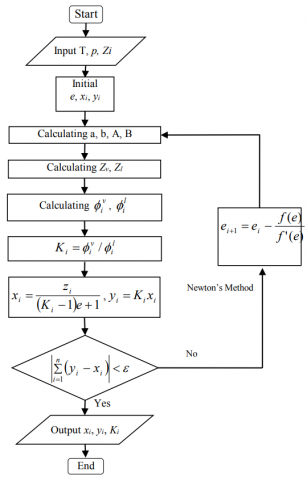Figure 1. Calculation flow chart for phase equilibrium

This paper writes Visual-Basic statements align with Newton’s Method based on the calculation idea above. It can calculate the gas and liquid distribution and their ration. The key steps for gasification rate calculation is shown as follow:

e0=0.5

K(i) = hv(i) / hl(i)

x(i) = Z(i) / (2 * (1 - e))

y(i) = Z(i) / (2 * e)

For j = 1 To 3

fun(j) = Z(j) * (K(j) - 1) * (1 - e + e * K(j))

dev(j) = -Z(j) * (K(j) - 1) ^ 2 / (1 - e + e * K(j)) ^ 2

fun = fun + fun(j)

dd = dev + dev(j)

Next j

If fun > 10 ^ (-6) Then

e = e - dev / fun

End If

3. Results and Discussion

3.1 Vapor- liquid distribution

In mixed-refrigerant cycle, the main components of mixture refrigerant is C1, C2, C3, C4, C5, N2, which is similar in composition to the natural gas after dehydration and deacidification. And the cooling curve (Q-T) of mixed-refrigerant is consistent with curve of natural gas. Hence, equilibrium calculation of mixture refrigerant and raw natural gas can be handled in the same way. Chose raw natural gas with simple components and calculate the vapor-liquid distribution at different conditions. Table 1 shows the basic thermodynamic parameters of each components . And Table 2 shows five different thermodynamic conditions.

Table 1.  Thermodynamic parameters of natural gas

 Components Formula M Tcr pcr w g/mol K ×10-5Pa methane CH4 16.042 190.69 46.04 0.013 ethane C2H6 30.068 305.38 48.80 0.102 propane C3H8 44.094 369.89 42.50 0.157 butane i-C4H10 58.120 408.13 36.48 0.183

Table 2.  Thermodynamic conditions for equilibrium calculation

 Components No.1 No.2 No.3 No.4 No.5 5.44   MPa 213.9 K 4.08 MPa 213.9 K 2.72 MPa 213.9 K 2.04 MPa 172.2 K 1.36 MPa 172.2 K C1 0.8450 0.7434 0.6199 0.9260 0.7619 C2 0.1476 0.2165 0.3231 0.0249 0.2036 C3 0.0074 0.0401 0.057 0.0491 0.0345

SRK equation and PR equation are used to obtain the vapor-liquid distribution and phase equilibrium constant at five conditions, as shown in Table 2. The calculated values predicted by the equation and compared with experimental data  in Figure 2. Also shown in figure 2 are a good agreement between them. The %ADD of vapor fraction was found to be 4.90% and 4.78% by using SRK-EOS and PR-EOS respectively, and the %ADD of liquid fraction are 6.27% and 6.26%, respectively. The SRK and PR EOSs predict the equilibrium constant with an average %ADD of 8.81% and 8.20%, respectively. It is worth considering that the RKS and PR EOSs can predict the equilibrium of LNG mixtures with a high precision and PR-EOS is with smaller error, especially. And the precision is nearly independent of the liquefaction condition in the case of mid & low-pressure. Nevertheless these two equations give a better agreement between calculated VLE and published experimental values.

## 2a.png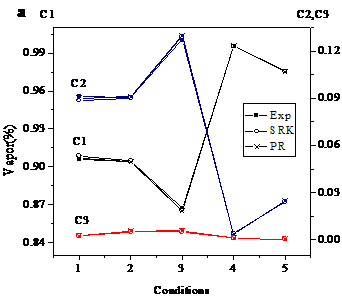## 2b.png## 2c.png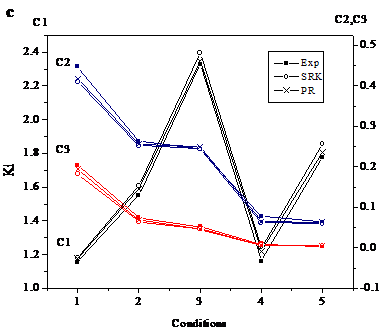Figure 2. Comparison between calculated values and experimental data by SRK and PR EOSs (a) gas mole fraction; (b) liquid mole fraction; (c) equilibrium constant

3.2 Optimization of equilibrium constants

Vapor-liquid phase equilibrium constant (yi/xi) can describe the phase distribution of each components for the liquefaction process clearly. Analysis of equilibrium constant for raw natural gas provides a basis for calculation of enthalpy and entropy in total process, and it is a good response of the liquefaction degree and energy consumption, which helps to real-time control for natural liquefaction. On the other hand, equilibrium calculation of mixture refrigerant can be used to know the cooling efficiency. Knowing from the previous section, in natural gas liquefaction technology, mixture composition, gas pressure and temperature are the main influence parameters on equilibrium. Because of the interaction effects between the three, parameters optimization calculation goes one-by-one assuming two of them are constants. In this section, equilibrium constants are calculated by PR equation of state, which gives a more accurate result than SRK equation. Vapor-liquid equilibrium constant of each components and gas-liquid ration of mixture system are shown as Figure 3-5.

## 3a.png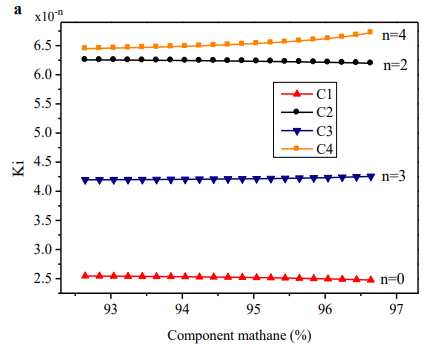## 3b.pngFigure 3. (a)Effects of component methane on equilibrium constant; (b)Effects of component methane on vapor-liquid ration

In Figure 3a, equilibrium constant of C1 will decrease with the increasing of C1 component, at the same time, the C4 constant will gradually increase and C2, C3 constant is relatively stable. As Figure 3b shown, vapor-liquid ration increases gradually. The more C1 component, the faster the gas-liquid ration increase since light hydrocarbon liquefaction is more difficult. Hence increasing C1, mixture system with C1-based will be difficult to liquefied. Then in equilibrium liquid phase decreasing lead to the constant increasing.

## 4a.png## 4b.png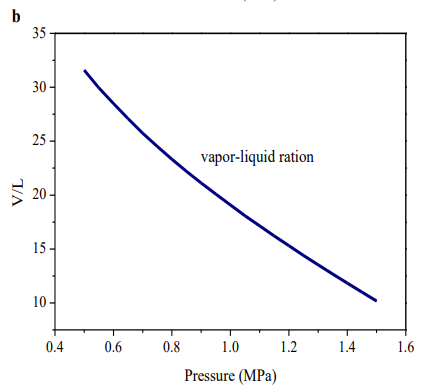Figure 4. (a)Effects of pressure on equilibrium constant; (b)Effects of pressure on vapor-liquid ration

At mid & low-pressure, the boiling point of each component is increasing with the increasing of pressure, which makes gasification more difficult and liquid phase increasing. For instance, equilibrium constant decreased. Given the complexity of propane and butane structures, equilibrium constant of C3 and C4 first decreased, then increased when the pressure is about 1.2MPa and 1.05MPa, respectively. Because C3 and C4 account for a small proportion in mixture, their particularity will not affect the general trend. And vapor-liquid ration of this system will lower when pressure increasing, with an almost linear relationship. Shown as Figure. 4a, 4b.

## 5a.png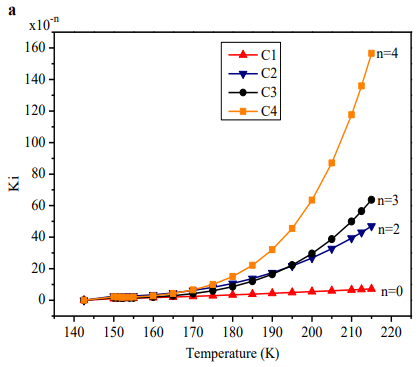## 5b.png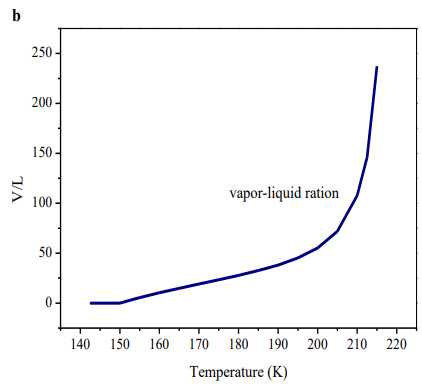Figure 5. (a) Effects of temperature on equilibrium constant; (b) Effects of temperature on vapor-liquid ration

Temperature shows a rather complicated influence on equilibrium constant, which needs take critical temperature and liquefaction temperature of natural gas into consideration. Natural gas cannot be liquefied when temperature is higher than critical temperature and no vapor is in existence below liquefied temperature. In proper temperature range, the responses of temperature rise on the increase of equilibrium constant are week, as appears in Figure 5. The boiling point of each components reduced when temperature rises, and gas phase species increased slightly. It caused equilibrium constant of all components increased. Especially near critical temperature, the constant increased sharply and a value zero of liquid ration were arrived. Below 150K, near the liquefaction temperature, vapor mole goes to zero as does equilibrium constant. Vapor-liquid ration of mixture has the same trend.

For raw natural gas, the lower the equilibrium constant, the more the liquid mole at liquid-vapor equilibrium state, which means a higher liquefaction degree and resource utilization. It is necessary for LNG project. And for refrigerant, the main component providing cold is liquid after separation, cooling, throttling, reducing pressure and temperature. In liquefaction process, the more the liquid mixtures there is in the refrigerant, the more heat of natural gas can be exchanged. And it means a higher utilization of mixture refrigerant. Hence smaller constant of raw natural gas and refrigerant is needed in liquefaction technology. The rational mixing ration of refrigerant with smaller lighter hydrocarbons, pressure increasing and temperature decreasing in critical and liquefied temperature range help optimization of liquefaction process.

4. Conclusions

Phase equilibrium is a basis of calculation of enthalpy and entropy, and it is a clearly reveal of liquefied degree of raw natural gas and cooling efficiency of mixture refrigerant. Using RKS and PR, two constants and cubic equation of state, a relatively accurate vapor-liquid distribution can obtained in the natural gas liquefaction process with mixed refrigerant , which will serve as a basic theories for liquefaction simulation. Comparing the two equation, PR gained more accurate results. According to PR-EOS calculation of impact factors on technology parameters, in mixing refrigerant cycle raw natural gas and mixture refrigerant need to keep a lower vapor-liquid constant. The rational mixing ration with less light hydrocarbons, pressure increasing and temperature decreasing in critical and liquefied temperature range can decrease vapor-liquid ration. It helps to reduce energy consumption, improve cooling efficiency and optimizing liquefaction technology.

Acknowledgement

The authors gratefully expressed their thanks for the financial support for these researches from the Foundation of State Key Laboratory of Coal Combustion (no.FSK LCC1210), from the National Natural Science Foundation of China (no. 51306022, 51176059) and from the National Natural Science Foundation of Hubei Province (no. 2013CFB398).

Nomenclature
 p pressure(Pa) T temperature(K) Vm molar volume(m3/mole) R molar gas constant, R=8.3145J/(mol.K) wi acentric factor a,b, m related constants of EOSs xi liquid molar fraction yi gas molar fraction zi molar fraction Tc,i critical temperature pc,i critical pressure kij binary interaction coefficient Ki equilibrium constantvapor fugacity coefficientliquid fugacity coefficient Superscripts V vapor L liquid Subscripts i component; The i-th j component; The j-th c critial
References

1. Zheng Y., Xia D., Liu X., Li J. Influence factors analysis of propane-mixed refrigerant natural gas liquefaction process based on HYSYS software model. Chemical Engineering of Oil and Gas. 2013, 42(5):473- 477.

2. Ho-Myung Chang; Jae Hoon Park;Kyung Hyun Gwak;Kun Hyung Choe. Nitrogen expander Cycles for Large Capacity Liquefaction of Natural Gas. AIP Conference Proceedings. 2014, 1573:1652-1657.

3. Wang, Meiqian;Zhang, Jian;Xu, Qiang. Optimal design and operation of a C3MR refrigeration system for natural gas liquefaction. Computers & Chemical Engineering. 2012, 39: 84-95.

4. Ho-Myung Chang, Hye Su Lim;Kun Hyung Choe. Effect of multi-stream heat exchanger on performance of natural gas liquefaction with mixed refrigerant. Cryogenics. 2012, 52(12): 642-647.

5. Ji-Hyun Hwang, Myung-Il Roh, Kyu-Yeul Lee. Determination of the optimal operating conditions of the dual mixed refrigerant cycle for the LNG FPSO topside liquefaction process. Computers & Chemical Engineering. 2013, 49: 25-36.

6. L. Castillo, M. Majzoub Dahouk, S. Di Scipio, C.A. Dorao. Conceptual analysis of the precooling stage for LNG processes. Energy Conversion and Management. 2013,66:41-47.

7. Amir Mortazavi, Abdullah Alabdulkarem, Yunho Hwang, Reinhard Radermacher. Novel combined cycle configurations for propane pre-cooled mixed refrigerant (APCI) natural gas liquefaction cycle. Applied Energy. 2014: 76-86.

8. Li J. Wu H. S. Equilibrium calculation of liquefaction natural gas. Gas and Heat. 2007, 27(10).

9. Li P. M. Jiao W. L. Phase equilibrium calculation in natural gas liquefaction with PR equation. Gas and Heat. 2008, 28(4).

10. Kh. Nasrifar, M. Moshfeghian. Vapor–liquid equilibria of LNG and gas condensate mixtures by the NasrifarMoshfeghian equation of state. Fluid Phase Equilibria, 2001, 200:203-216.

11. Ali Haghtalab, Peyman Mahmoodi, Seyed Hossein Mazloumi. A modified Peng-Robinson equation of state for phase equilibrium calculation of liquefied, synthetic natural gas, and gas condensate mixtures. The Canadian Journal of Chemical Engineering. 2011, 89.

12. Paolo Stringari; Marco Campestrini; Christophe Coquelet; Philippe Arpentinier. An equation of state for solid–liquid–vapor equilibrium applied to gas processing and natural gas Liquefaction. Fluid Phase Equilibria. 2014, 362:258-267.

13. Soave, G. Equilibrium constants from a modified Redlich-Kwong equation of state. Chem Eng Science, 1972, 27: 1197-1203.

14. Peng, D.Y.; Robinson, D.B. A new two-constant equation of state. Ind. Eng. Chem. Fundamment, 1976, 15:59-64.

15. K. S. Pedersen, Aa. Fredenslund, P. Thomassen. Contributions in petroleum gelogy and engineering properties of oils and natural gases. Gulf Publishing Company. 1989.

16. Ma P. S.Chemical engineering thermodynamics. Chemical Industry Press. Beijing, 2005, 5.

17. Gu A. Z. Technology of LNG. China Machine Press. Beijing, 2003, 10.

18. Richard E. S.; Claus, B.; Gordon, J. V. W. Fundamentals of Thermodynamics. 6th ed; John Wiley & Sons Inc: New York, 2003, 656.

19. M. Mukhopadhyay, R. Awashi. K-value predictions for the methane-ethane-propane system. Cryogenics, 1981, 6.